# Maximum and minimum facts for kids

Kids Encyclopedia Facts

In mathematics, the maximum and minimum of a set A is the largest and smallest element of A. They are written as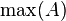$\max(A)$ and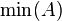$\min(A)$, respectively. Similarly, the maximum and minimum of a function are the largest and smallest value that the function takes at a given point. Together, they are known as the extrema (the plural of extremum).

Minimum means the least you can do of something. For example, if the minimum amount of dollars you must pay for something is seven, then you cannot pay six dollars or less (you must pay at least seven). You can do more than the minimum, but no less. Maximum means the most you can have of something. For example, if the maximum amount of oranges you can juggle is five, you cannot juggle more than five oranges. You can do the maximum or less.

For a differentiable function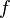$f$, if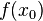$f(x_0)$ is an extreme value for the set of all values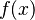$f(x)$, and if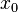$x_0$ is in the interior of the domain of$f$, then$x_0$ is a critical point, by Fermat's theorem.

## Images for kidsMaximum and minimum Facts for Kids. Kiddle Encyclopedia.